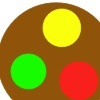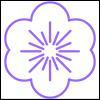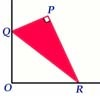# Search by Topic

Filter by: Content type:
Age range:
Challenge level:

### There are 50 results

Broad Topics > Angles, Polygons, and Geometrical Proof > Circle properties and circle theorems##### Age 11 to 16 Challenge Level:

Draw some quadrilaterals on a 9-point circle and work out the angles. Is there a theorem?##### Age 14 to 16 Challenge Level:

Explore when it is possible to construct a circle which just touches all four sides of a quadrilateral.### Partly Circles

##### Age 14 to 16 Challenge Level:

What is the same and what is different about these circle questions? What connections can you make?### The Line and Its Strange Pair

##### Age 14 to 16 Challenge Level:

In the diagram the point P' can move to different places along the dotted line. Each position P' takes will fix a corresponding position for P. If P' moves along a straight line what does P do ?### Right Angles

##### Age 11 to 14 Challenge Level:

Can you make a right-angled triangle on this peg-board by joining up three points round the edge?### Subtended Angles

##### Age 11 to 14 Challenge Level:

What is the relationship between the angle at the centre and the angles at the circumference, for angles which stand on the same arc? Can you prove it?### Triangles in Circles

##### Age 11 to 14 Challenge Level:

Can you find triangles on a 9-point circle? Can you work out their angles?### Salinon

##### Age 14 to 16 Challenge Level:

This shape comprises four semi-circles. What is the relationship between the area of the shaded region and the area of the circle on AB as diameter?### Belt

##### Age 16 to 18 Challenge Level:

A belt of thin wire, length L, binds together two cylindrical welding rods, whose radii are R and r, by passing all the way around them both. Find L in terms of R and r.### Lens Angle

##### Age 14 to 16 Challenge Level:

Find the missing angle between the two secants to the circle when the two angles at the centre subtended by the arcs created by the intersections of the secants and the circle are 50 and 120 degrees.### Sitting Pretty

##### Age 14 to 16 Challenge Level:

A circle of radius r touches two sides of a right angled triangle, sides x and y, and has its centre on the hypotenuse. Can you prove the formula linking x, y and r?### Compare Areas

##### Age 14 to 16 Challenge Level:

Which has the greatest area, a circle or a square inscribed in an isosceles, right angle triangle?### Baby Circle

##### Age 16 to 18 Challenge Level:

A small circle fits between two touching circles so that all three circles touch each other and have a common tangent? What is the exact radius of the smallest circle?### Sports Equipment

##### Age 7 to 11 Challenge Level:

If these balls are put on a line with each ball touching the one in front and the one behind, which arrangement makes the shortest line of balls?### Perfect Eclipse

##### Age 14 to 16 Challenge Level:

Use trigonometry to determine whether solar eclipses on earth can be perfect.##### Age 14 to 16 Challenge Level:

Investigate the properties of quadrilaterals which can be drawn with a circle just touching each side and another circle just touching each vertex.### Circle Theorems

##### Age 14 to 16 Challenge Level:

This set of resources for teachers offers interactive environments which support work on properties of angles in circles at Key Stage 4.### Interacting with the Geometry of the Circle

##### Age 5 to 16

Jennifer Piggott and Charlie Gilderdale describe a free interactive circular geoboard environment that can lead learners to pose mathematical questions.### Mapping the Wandering Circle

##### Age 14 to 16 Challenge Level:

In the diagram the point P can move to different places around the dotted circle. Each position P takes will fix a corresponding position for P'. As P moves around on that circle what will P' do?### Circle Scaling

##### Age 14 to 16 Challenge Level:

Describe how to construct three circles which have areas in the ratio 1:2:3.### Circle Box

##### Age 14 to 16 Challenge Level:

It is obvious that we can fit four circles of diameter 1 unit in a square of side 2 without overlapping. What is the smallest square into which we can fit 3 circles of diameter 1 unit?### Flower

##### Age 16 to 18 Challenge Level:

Six circles around a central circle make a flower. Watch the flower as you change the radii in this circle packing. Prove that with the given ratios of the radii the petals touch and fit perfectly.### Lunar Angles

##### Age 16 to 18 Challenge Level:

What is the sum of the angles of a triangle whose sides are circular arcs on a flat surface? What if the triangle is on the surface of a sphere?### Circles in Circles

##### Age 16 to 18 Challenge Level:

This pattern of six circles contains three unit circles. Work out the radii of the other three circles and the relationship between them.### Arrh!

##### Age 14 to 16 Challenge Level:

Triangle ABC is equilateral. D, the midpoint of BC, is the centre of the semi-circle whose radius is R which touches AB and AC, as well as a smaller circle with radius r which also touches AB and AC. . . .##### Age 14 to 16 Challenge Level:

The sides of a triangle are 25, 39 and 40 units of length. Find the diameter of the circumscribed circle.### Incircles Explained

##### Age 16 to 18##### Age 11 to 16

This gives a short summary of the properties and theorems of cyclic quadrilaterals and links to some practical examples to be found elsewhere on the site.### Crescents and Triangles

##### Age 14 to 16 Challenge Level:

Can you find a relationship between the area of the crescents and the area of the triangle?### Similarly So

##### Age 14 to 16 Challenge Level:

ABCD is a square. P is the midpoint of AB and is joined to C. A line from D perpendicular to PC meets the line at the point Q. Prove AQ = AD.### Tricircle

##### Age 14 to 16 Challenge Level:

The centre of the larger circle is at the midpoint of one side of an equilateral triangle and the circle touches the other two sides of the triangle. A smaller circle touches the larger circle and. . . .### Three Balls

##### Age 14 to 16 Challenge Level:

A circle has centre O and angle POR = angle QOR. Construct tangents at P and Q meeting at T. Draw a circle with diameter OT. Do P and Q lie inside, or on, or outside this circle?##### Age 14 to 16 Challenge Level:

A picture is made by joining five small quadrilaterals together to make a large quadrilateral. Is it possible to draw a similar picture if all the small quadrilaterals are cyclic?### Encircling

##### Age 14 to 16 Challenge Level:

An equilateral triangle is sitting on top of a square. What is the radius of the circle that circumscribes this shape?### Three Four Five

##### Age 14 to 16 Challenge Level:

Two semi-circles (each of radius 1/2) touch each other, and a semi-circle of radius 1 touches both of them. Find the radius of the circle which touches all three semi-circles.### Tied Up

##### Age 14 to 16 Short Challenge Level:

How much of the field can the animals graze?### Not So Little X

##### Age 11 to 14 Challenge Level:

Two circles are enclosed by a rectangle 12 units by x units. The distance between the centres of the two circles is x/3 units. How big is x?### Coins on a Plate

##### Age 11 to 14 Challenge Level:

Points A, B and C are the centres of three circles, each one of which touches the other two. Prove that the perimeter of the triangle ABC is equal to the diameter of the largest circle.### Escriptions

##### Age 16 to 18 Challenge Level:

For any right-angled triangle find the radii of the three escribed circles touching the sides of the triangle externally.### Strange Rectangle

##### Age 16 to 18 Challenge Level:

ABCD is a rectangle and P, Q, R and S are moveable points on the edges dividing the edges in certain ratios. Strangely PQRS is always a cyclic quadrilateral and you can find the angles.### Long Short

##### Age 14 to 16 Challenge Level:

What can you say about the lengths of the sides of a quadrilateral whose vertices are on a unit circle?### Circumspection

##### Age 14 to 16 Challenge Level:

M is any point on the line AB. Squares of side length AM and MB are constructed and their circumcircles intersect at P (and M). Prove that the lines AD and BE produced pass through P.### Medallions

##### Age 14 to 16 Challenge Level:

Three circular medallions fit in a rectangular box. Can you find the radius of the largest one?### Triangle Incircle Iteration

##### Age 14 to 16 Challenge Level:

Keep constructing triangles in the incircle of the previous triangle. What happens?### Incircles

##### Age 16 to 18 Challenge Level:

The incircles of 3, 4, 5 and of 5, 12, 13 right angled triangles have radii 1 and 2 units respectively. What about triangles with an inradius of 3, 4 or 5 or ...?### Just Touching

##### Age 16 to 18 Challenge Level:

Three semi-circles have a common diameter, each touches the other two and two lie inside the biggest one. What is the radius of the circle that touches all three semi-circles?### Set Square

##### Age 16 to 18 Challenge Level:

A triangle PQR, right angled at P, slides on a horizontal floor with Q and R in contact with perpendicular walls. What is the locus of P?### Kissing

##### Age 16 to 18 Challenge Level:

Two perpendicular lines are tangential to two identical circles that touch. What is the largest circle that can be placed in between the two lines and the two circles and how would you construct it?### Fixing It

##### Age 16 to 18 Challenge Level:

A and B are two fixed points on a circle and RS is a variable diamater. What is the locus of the intersection P of AR and BS?### Some(?) of the Parts

##### Age 14 to 16 Challenge Level:

A circle touches the lines OA, OB and AB where OA and OB are perpendicular. Show that the diameter of the circle is equal to the perimeter of the triangle